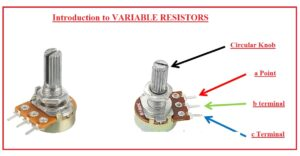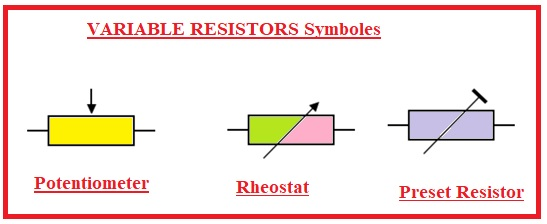Hello, readers welcome to new post. Today we will learn HOW VARIABLE RESISTORS WORK. The main part of electric circuitry is inserted as resistances. There are different types of resistance that exist and that are employed in different applications. Commonly used types of resistance are fixed variables in the basis of their resistive value.

In this post, we will cover the basics of Variable resistors working features and some other points. So let’s start with HOW VARIABLE RESISTORS WORK

### Introduction to VARIABLE RESISTORS

• The variable resistor is a category of resistance that varies resistance from 0 to a specific value. Its common applications are for voltage regulation.
• Its function is that as the value of resistance is increased for this module current flow decreases.
• it is an electro-mechanical transducer and works through slider contact at the resistive component.
• If it is used as potential dividers through the implementation of 3 points named potentiometer.
• In the case of two points work as a variable resistance and is called a rheostat.
• There is electronically implemented resistors are used called digital potentiometers#### Definition of  Variable Resistor

• The variable resistor also called a potentiometer or rheostat that is used in circuits for resistance change. It is a passive component and comes with 3 pins, through this component value of resistance can be varied according to changes in the position of the moving wiper existing on the resistor.
• These resistors are commonly used in circuits to control current or voltage in the circuit and it also varies the amplifier gain or control brightness of LED or Displays. It has different sizes and structures from small-size components to larger panel mount potentiometers#### VARIABLE RESISTORS Symboles

• Here you can see symobls of variable resistors### What is a variable resistance

• Variable resistance is a type of electrical component that allows for the adjustment of its resistance value. This property makes variable resistors a versatile tool in a wide variety of applications. It is a commonly used resistor in circuits where it controls the current flow in the circuitry
• The common use of variable resistors audio circuits. Potentiometer is a variable resistor used in speakers to control the volume of sound, headphones, and other audible devices. By adjusting potentiometer resistance we can adjust the volume of the audio signal.
• This variable resistor is used in dimmer switches. Adjusting the resistance brightness of the light can be controlled.
• In experimental setups where differnt value of resistance is required variable resistors are used. For example, In physical experiments, engineers can vary the circuit resistance to control current flow.

### Construction of a Variable Resistor

• This resistor has a resistive component and a moveable wiper that move on the resistive element. The resistive element can be in the shape of a thin layer deposited on a ceramic or plastic substrate. The resistive elements can be varied through change the length or thickness of resistive material or by varying the pitch of wire also wire dia
• The fixed terminal are mostly created with metallic substances like copper or brass and attached at endpoints of resistive elements.
• Wiper has a connection with one fixed terminal and the resistance value between the wiper and other fixed pints changes with the movement of the wiper
• Variables resistors can be designed in differnt shapes and sizes on the base of users. These resistors are designed to use in PCB boards and also for panel mounting.  They come with differnt resistance values power ratings and other features according to use.### Operation Principles of Variable Resistors

• The working principle of variable resistors is based on the change in resistance by adjusting the position of the moveable wiper across resistive components.  The resistive component is created with the use of carbon, wire wound, or conductive plastic.
• During the movement of the wiper, the resistive component creates a new electrical path among the two fined points of the resistor, which varies the resistance value among these two points. If the resistance value is increased current in the circuit will decrease and if the resistance is reduced current in the circuit will be increased.
• The relation between the wiper position and the resistance value is linear or logarithmic on the base of the variable resistor type. For linear resistors, the resistance value varies in proportional relation to the wiper position, and in the case of the logarithmic variable resistor the resistance value varies in a logarithmic way.

### Types of Variable Resistances

Type Description Example Application
Single-Turn can be changed by turning a slider or a knob all the way around. Typically range from a few ohms to a few kiloohms in resistance. Timer circuits, oscillator circuits, oscillator gain control, and bias adjustment.
Multi-Turn Although they have a wider range of resistance values (up to several megaohms), they are similar to single-turn variable resistors. can be changed by turning a knob a number of times. calibration of test and measurement equipment, electronic circuit fine-tuning, and radio frequency tuning.
Linear With respect to the wiper’s location, resistance changes linearly.
.
Gain control, voltage regulators, tone control, and volume control.
Logarithmic The resistance varies logarithmically as the wiper moves. Gain control, voltage regulators, and tone controls for audio. For audio applications, logarithmic potentiometers are recommended because they offer a more consistent attenuation of sound.
.
Trimmer During assembly or production, small, predetermined variable resistors are utilized to change the resistance of a circuit. calibration of test and measurement equipment, as well as electrical circuit fine-tuning. When a precise, set resistance value is required, trimmers are utilized.
Digital Digital variable resistors modify the resistance value using a sequence of switches or relays as opposed to a wiper. robotics, motor control, audio equipment, and digital-to-analog converters.

### Types of Variable Resistors

• There are three main types of variable resistors are discussed here
• Potentiometers
• Rheostats
• Digital potentiometers### What is Potentiometer

• For the potentiometer to change the resistance in circuitry rotary knob is used. There are three pinouts are configured on the potentiometer.
• Among the 2 pins configured on the outer side, their resistive element exists used for resistance changes.
• A central pinout is used as a wiper. This wiper is configured among the 2 endpoints.
• Ponts can be moved to the connection resistive elements by operating the shaft of the potentiometer
• If we move the wiper or slider to the left side decrement in the resistance among the central pins and left-positioned pin. So resistance among the central pin and right pinout increases when the slider moves to left

#### POTENTIOMETERS as VOLTAGE DIVIDERS

• The voltage divider is circuitry used for the reduction of volts in circuitry. The output volts rely at the ratio of 2 resistance linked in a series combination.
• Output are get among the 2 resistances. The formula for voltage dividers to measure the volts is given
• Vout= R2/(R1+R2) x Vin
• Ri is the resistance nearest to input volts and R2 is the resistance near to the ground. Vin is the input and Vout is the output volts

## potentiometer vs variable resistor

 Function Potentiometer is used as a voltage divider or to adjust the resistance of circuitry. Variable resistors are Used to adjust the resistance of a circuit Construction it comes with a resistive element and a sliding contact that moves along the element It comprises of the resistive element and a movable wiper that can be adjusted to the resistance change Types Its main types are Linear potentiometer and  logarithmic potentiometer Its common types are single-turn variable resistors and multi-turn variable resistor Resistance Range Generally few ohms to several megaohms Normally few ohms to several kiloohms Tolerance ±5% or ±10% ±5% or ±10% Temperature Coefficient it can be high or low on the basis of type It can be low or high according to the type Power Rating it can handle high powers values about several watts) It handles low power ratings (usually up to 1 watt) Mechanical Life It is sensitive to vibration and wear it is more durable than potentiometers Price It is more costly than variable resistors It is less costly than potentiometers Applications tone control, Volume control,  variable gain circuits, voltage regulators gain control, timer circuits Bias adjustment,, oscillator circuits Advantages It provides accurate  control of resistance and voltage and can be used as a voltage divider Easy to use and adjust, less sensitive to wear and vibration Disadvantages It has sensitive nature to wear and vibration, more expensive than variable resistors it has a Limited range of resistance, less accurate than potentiometers

#### DIGITAL POTENTIOMETERS

• This potentiometer used digital signals to vary the resistance in place of mechanical movement for resistance change.
• Digital potentiometers vary resistance steps-wise on the basis of the digital signal moving through it. It is used for applications where vibration, dust, and moisture.

Rheostat

• The physical structure of rheostat are like the potentiometers and the difference is that rheostat is not used as potential dividers but work as variable resistance
• It works on the 2 terminals in place of three point-like potentiometers
• Its one point is connected at the resistive component and the second at the wiper of variable resistance.
• It has been used for power regulation circuits in series combinations like a light bulb.
• Currently not used for power control circuits since power losses occur
• In power regulation applications rheostat is replaced with switching circuits.

That is all about the HOW VARIABLE RESISTORS WORK. All details has explained if you ave any further queries ask in the comments,

### 1 Comment

1.Donny says:

very detailed explained